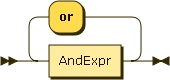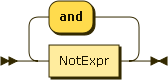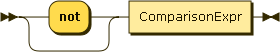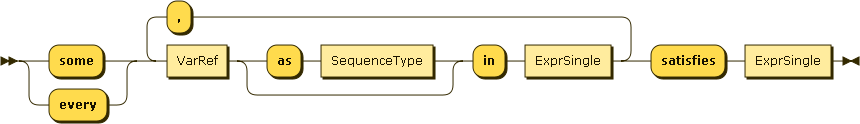### Logics

JSONiq follows the W3C standard for logical expressions; it introduces a prefix unary not operator as a synonym for fn:not, and extends the semantics of effective boolean values to objects, arrays and nulls. The following explanations, provided as an informal summary for convenience, are non-normative.

Figure 32. OrExprFigure 33. AndExprFigure 34. NotExprJSONiq logics support is based on two-valued logics: just true and false.

Non-boolean operands get automatically converted to either true or false, or an error is raised. The boolean() function performs a manual conversion.

• An empty sequence is converted to false.

• A singleton sequence of one null is converted to false.

• A singleton sequence of one string is converted to true except the empty string which is converted to false.

• A singleton sequence of one number is converted to true except zero or NaN which are converted to false.

• An operand singleton sequence whose first item is an object or array is converted to true.

• Other operand sequences cannot be converted and an error is raised.

JSONiq supports the most famous three boolean operations: conjunction, disjunction and negation. Negation has the highest precedence, then conjunction, then disjunction. Parentheses can override.

Example 53. Logics with booleans

```true and ( true or not true )
```

Result (run with Zorba): true

Example 54. Logics with comparing operands

```1 + 1 eq 2 or 1 + 1 eq 3
```

Result (run with Zorba): true

Example 55. Conversion of the empty sequence to false

```boolean(())
```

Result (run with Zorba): false

Example 56. Conversion of null to false

```boolean(null)
```

Result (run with Zorba): false

Example 57. Conversion of a string to true

```boolean("foo"), boolean("")
```

Result (run with Zorba): true false

Example 58. Conversion of a number to false

```0 and true, not (not 1e42)
```

Result (run with Zorba): false true

Example 59. Conversion of an object to a boolean (not implemented in Zorba at this point)

```{ "foo" : "bar" } or false
```

Result (run with Zorba): true

If the input sequence has more than one item, and the first item is not an object or array, an error is raised.

Example 60. Error upon conversion of a sequence of more than one item, not beginning with a JSON item, to a boolean

```( 1, 2, 3 ) or false
```

Result (run with Zorba): An error was raised: invalid argument type for function fn:boolean(): effective boolean value not defined for sequence of more than one item that starts with "xs:integer"

Unlike in C++ or Java, you cannot rely on the order of evaluation of the operands of a boolean operation. The following query may return true or may return an error.

Example 61. Non-determinism in presence of errors.

```true or (1 div 0)
```

Result (run with Zorba): true

JSONiq follows the W3C standard for quantified expressions. The following explanations, provided as an informal summary for convenience, are non-normative.

Figure 35. QuantifiedExprIt is possible to perform a conjunction or a disjunction on a predicate for each item in a sequence.

Example 62. Universal quantifier

```every \$i in 1 to 10 satisfies \$i gt 0
```

Result (run with Zorba): true

Example 63. Existential quantifier on several variables

```some \$i in -5 to 5, \$j in 1 to 10 satisfies \$i eq \$j
```

Result (run with Zorba): true

Variables can be annotated with a type. If no type is specified, item* is assumed. If the type does not match, an error is raised.

Example 64. Existential quantifier with type checking

```some \$i as integer in -5 to 5, \$j as integer in 1 to 10 satisfies \$i eq \$j
```

Result (run with Zorba): true